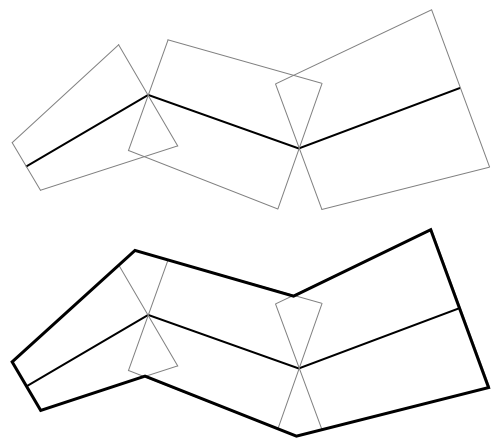2021.1.14

• All Superinterfaces:
java.lang.Cloneable, ILcdBounded, ILcdCloneable, ILcdShape, java.io.Serializable
All Known Subinterfaces:
ILcd2DEditableVariableGeoBuffer, ILcd3DEditableVariableGeoBuffer
All Known Implementing Classes:
TLcdLonLatHeightVariableGeoBuffer

```public interface ILcdVariableGeoBuffer
extends ILcdShape```
An `ILcdVariableGeoBuffer` shape defines a buffer or corridor around a given (3D) polyline, adding a width and height to the shape that is defined for every polyline point.

In 2D, the contour of the buffer is a polygon in which each exterior point lies at the relevant `distance` from the line segments of the `polyline`.
In 3D, the contour can be seen as the 2D contour, extruded along the Z-axis. However, the altitude and height of the contour at each point can be different.This images shows how the 2D contour of the variable geo buffer is defined. Each segment of the axis point list (and the corresponding widths of the axis points) defines a isosceles trapezoid. These trapezoids are then connected with each by calculating the intersections between their edges.

Since:
11.1
`ILcdGeoBuffer`
• ### Method Summary

All Methods
Modifier and Type Method and Description
`ILcdPointList` `getBaseShape()`
Returns the base polyline of the buffer.
`double` `getHeightAbove(int aIndex)`
Returns the height of this buffer above the base polyline point with the given index, expressed in meters.
`double` `getHeightBelow(int aIndex)`
Returns the height of this buffer below the base polyline point with the given index, expressed in meters.
`double` `getWidth(int aIndex)`
Returns the width of this buffer at the given point index, expressed in meters.
• ### Methods inherited from interface com.luciad.shape.ILcdShape

`contains2D, contains2D, contains3D, contains3D, getFocusPoint`
• ### Methods inherited from interface com.luciad.shape.ILcdBounded

`getBounds`
• ### Methods inherited from interface com.luciad.util.ILcdCloneable

`clone`
• ### Method Detail

• #### getWidth

```double getWidth(int aIndex)
throws java.lang.IndexOutOfBoundsException```
Returns the width of this buffer at the given point index, expressed in meters. This width is defined as the distance from the respective point in the base polyline, in a direction perpendicular to the segments of the base polyline containing the respective point. This is also illustrated in the class doc of this interface.
Parameters:
`aIndex` - the base shape point index to return the width for
Returns:
a positive integer (>= 0) representing the width of the buffer at the given point
Throws:
`java.lang.IndexOutOfBoundsException` - if the base polyline does not have a point for the given index
• #### getHeightBelow

```double getHeightBelow(int aIndex)
throws java.lang.IndexOutOfBoundsException```
Returns the height of this buffer below the base polyline point with the given index, expressed in meters. This height is defined as the distance between the respective point and the lower side of the contour at that point.
Parameters:
`aIndex` - the base shape point index to return the height for
Returns:
a positive integer (>= 0) representing the distance between the respective point and the lower side of the contour at that point
Throws:
`java.lang.IndexOutOfBoundsException` - if the base polyline does not have a point for the given index
• #### getHeightAbove

```double getHeightAbove(int aIndex)
throws java.lang.IndexOutOfBoundsException```
Returns the height of this buffer above the base polyline point with the given index, expressed in meters. This height is defined as the distance between the respective point and the upper side of the contour at that point.
Parameters:
`aIndex` - the base shape point index to return the height for
Returns:
a positive integer (>= 0) representing the distance between the respective point and the upper side of the contour at that point
Throws:
`java.lang.IndexOutOfBoundsException` - if the base polyline does not have a point for the given index
• #### getBaseShape

`ILcdPointList getBaseShape()`
Returns the base polyline of the buffer.
Returns:
the base polyline of the buffer.